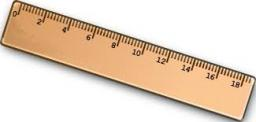# Express 4659

Express the ratio in the basic form.
a) 1cm: 1m
b) 3 ha: 30 a
c) 50 kg: 1 t
d) 2.5 m: 10 cm

a =  1/100
b =  10
c =  1/20
d =  25

### Step-by-step explanation:Did you find an error or inaccuracy? Feel free to write us. Thank you!

Tips for related online calculators
Need help calculating sum, simplifying, or multiplying fractions? Try our fraction calculator.
Do you want to convert length units?
Do you want to convert mass units?